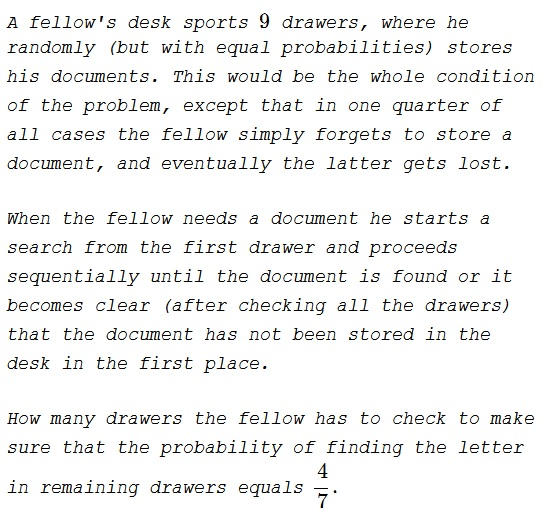# Diminishing Hopes II

### ProblemFive.

### Solution 1

Let's add three imaginary drawers to the desk and assume that this is where the documents get lost. We should add three drawers.

With $k$ drawers to go, of which two are unavailable, the probability of a successful outcome is $\displaystyle \frac{k - 3}{k}.$ With $\displaystyle k = 12 - n,$ this reduces to $\displaystyle P_{n} = \frac{9 - n}{12 - n}.$ This is a decreasing function of $n$ for $\displaystyle n < 12.$

We need $n$ such that $\displaystyle \frac{9 - n}{12 - n}=\frac{4}{7}$ from which $n=5.$

### Solution 2

\displaystyle \begin{align} &P(\text{finding in last }9-k|\text{not found in first }k) \times P(\text{not found in first }k)\\ &\qquad\qquad= P(\text{finding in last }9-k\text{ AND not found in first }k)\\ &\qquad\qquad= P(\text{finding in last }9-k). \end{align}

Hence, $\displaystyle \frac{4}{7}\times\left(1-\frac{k}{12}\right) = \frac{9-k}{12}$ from which $k=5.$

### Acknowledgment

This is an adaptation of an earlier problem Diminishing Hopes.

Solution 2 is by Amit Itagi.Copyright © 1996-2018 Alexander Bogomolny

 64859780